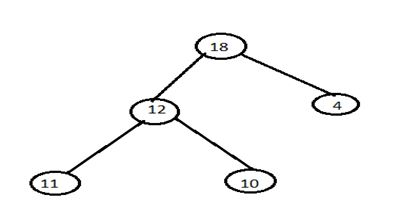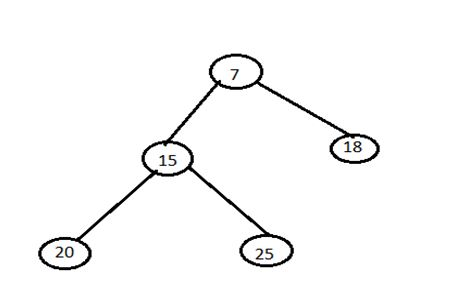# Heap Sort (Introduction, Algorithm and Program using C)

Learn: In this article we are going to study about Heap sort, Implementation of heap sort in C language and the algorithm for heap sort.
Submitted by Abhishek Kataria, on June 13, 2018

## Heap sort

Heap sort was invented by John Williams. Heap sort is a sorting technique of data structure which uses the approach just opposite to selection sort. The selection sort finds the smallest element among n elements then the smallest element among n-1 elements and so on. Until the end of the array heap sort finds the largest element and put it at end of the array, the second largest element is found and this process is repeated for all other elements

## What is Heap?

Heap is a complete binary tree in which every parent node be either greater or lesser than its child nodes. Heap can be of two types that are:

1) Max heap

A max heap is a tree in which value of each node is greater than or equal to the value of its children node.

Example of max heap:2) Min heap

A min heap is a tree in which value of each node is less than or equal to the value of its children node.

Example of min heap:### Working of Heap Sort

1. The heap sort begins by building a heap out of the data set and then removing the largest item and placing it at the end of the sorted array.
2. After removing the largest item, it reconstructs the heap and removes the largest remaining item and places it in the next open position from the open position from the end of the sorted array.
3. This is repeated until there is no item left in the heap and the sorted array is full.
4. Elementary implementation requires two arrays one to hold the heap and the other to hold the sorted elements.

### Algorithm for heap sort

Procedure 1:

`INSHEAP(TREE, N, HEAP):`
• A heap H with N elements is stored in the array TREE, and VALUE information is given.
• This procedure inserts ITEM as a new element of H.
• PTR gives the location of ITEM as it rises in the tree, and PAR denotes the location of the parent of VALUE.
1. [Add new node to H and initialize PTR]
Set N: N+1 and PTR: =N
2. [Find location to insert VALUE]
[Find location to insert VALUE]
3. Set PAR:=[PTR/2] {Location of parent node}
4. If VALUE <=TREE[PAR],Then:
Set TREE[PTR]:=VALUE ,and return
5. Set TREE[PTR]:=TREE[PAR]
6. Set PTR:=PAR
[End of step 2 loop]
7. [Assign VALUE as the root of H]
Set TREE:=VALUE
8. Return

Procedure 2:

`DELHEAP(TREE,N,VALUE):`
• A Heap H with N elements is stored in the array TREE.
• This procedure assigns the root TREE  of H to the variable VALUE and then reheaps the remaining elements.
• The variable LAST saves the value of the original last node of H.
• The pointer PTR, LEFT and RIGHT gives the location of LAST and its left and right children as LAST sinks in the tree.
1. Set VALUE:=TREE
2. Set LAST:=TREE[N] and N :=N-1
3. Set PTR:=1,LEFT:=2 and RIGHT:=3
4. Repeats steps 5 to 7 while RIGHT<=N:
5. If LAST >=TREE[LEFT] and LAST>=TREE [RIGHT], then:
Set TREE[PTR]:=LAST and return
6. If TREE [RIGHT]<=TREE[LEFT],then:
Set TREE[PTR]:=TREE[LEFT] and PTR:=LEFT
Else:
Set TREE[PTR]:=TREE[RIGHT] and PTR:=RIGHT
7. Set LEFT:=2*PTR and RIGHT:=LEFT+1
8. If LEFT=N and if LAST<TREE[LEFT],then: set PTR :=LEFT
9. Set TREE[PTR]:=LAST
10. Return

Algorithm

`HEAPSORT (A, N):`
• An array A with N elements is given.
• This algorithm sorts the element of A.
1. [Build a heap A ,using a procedure 1]
Repeat for J=1 to N-1
Call INSHEAP(A, J, A[J+1])
2. [sort A by repeatedly deleting the root of H, using procedure 2]
Repeat while N>1:
Call DELHEAP(A , N,VALUE)
Set A[n+1]:=value
3. Exit

### Performance of heap sort

Heap Sort has O(nlogn) time complexities for all the cases (best case, average case and worst case).

### Program for heap sort in C language

```#include<stdio.h>

void create(int []);

int main()
{
int heap,n,i,last,temp;

printf("Enter no. of elements:");
scanf("%d",&n);

printf("\nEnter elements:");
for(i=1;i<=n;i++)
scanf("%d",&heap[i]);

//create a heap
heap=n;
create(heap);

//sorting
while(heap > 1)
{
//swap heap and heap[last]
last=heap;
temp=heap;
heap=heap[last];
heap[last]=temp;
heap--;
}

//print sorted data
printf("\nArray after sorting:\n");
for(i=1;i<=n;i++)
printf("%d ",heap[i]);

return 0;
}

void create(int heap[])
{
int i,n;
n=heap; //no. of elements

for(i=n/2;i>=1;i--)
}

{
int j,temp,n,flag=1;
n=heap;

while(2*i<=n && flag==1)
{
j=2*i;    //j points to left child
if(j+1<=n && heap[j+1] > heap[j])
j=j+1;
if(heap[i] > heap[j])
flag=0;
else
{
temp=heap[i];
heap[i]=heap[j];
heap[j]=temp;
i=j;
}
}
}
```

Output

```    Enter no. of elements:5
Enter elements:12 8 46 3 7
Array after sorting:
7 8 12 23 46
```